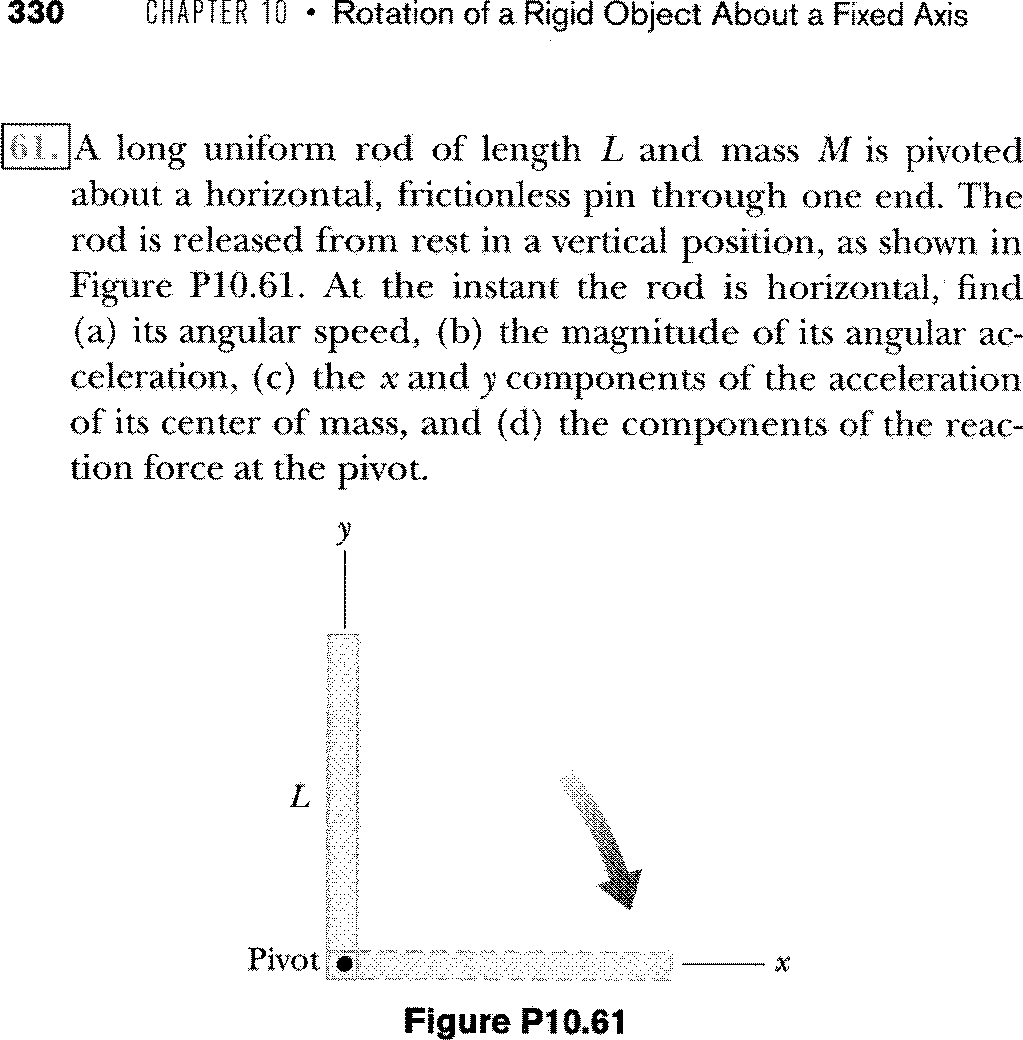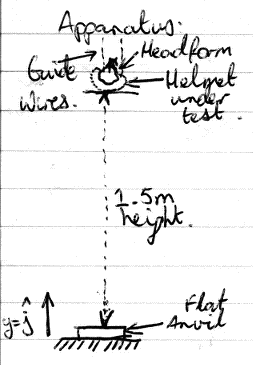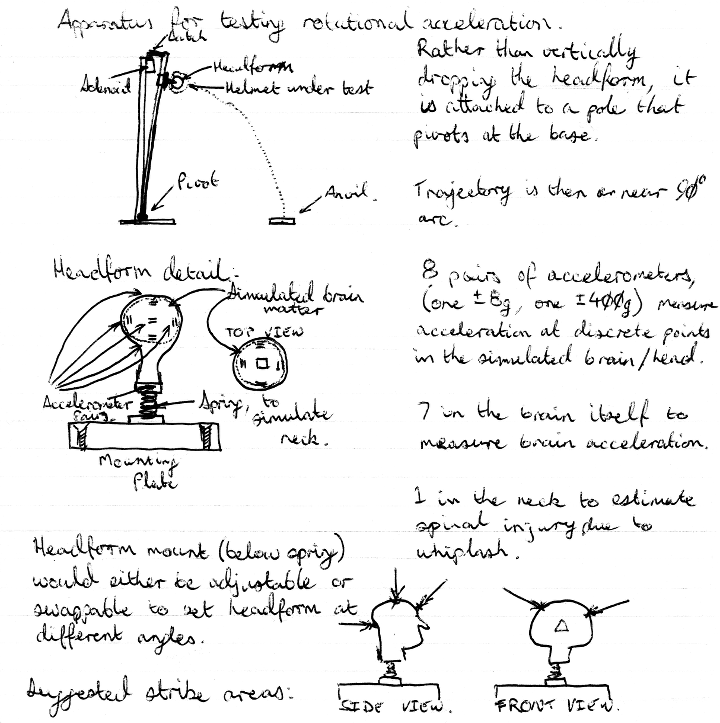As mentioned earlier, I’ve been doing a little “light” reading. Light reading being in the form of a 1500-page physics textbook which was purchased in my first year of engineering.

I’ve just been reading through it revising the material. Not doing the actual problems but just refreshing my memory, I figure I’ll go back and do selected problems once I’ve gone over everything.

I figured I’d see something that would perhaps jog my memory, or inspire me in one form or another.

One area was Hooke’s law, which relates the force exerted on a spring to its amount of displacement. I figure as a model of the brain, this might be one way to model it, not as a single mass, but as a series of “marbles”, if you will, connected by springs, as an analogy to the concept of neurons and their interconnections. The thought that, perhaps brains are not solid, but are a very dense mesh.

I’ll have to ponder this a bit more I guess. It’s basically a finite-element analysis approach to modelling the brain and what goes on inside. It’d be interesting to try a physical modelling of that mathematical model. Lead sinkers and strain gauges perhaps? I don’t know there.

The other was in relation to my test apparatus that I described earlier. In flicking through the problems, I found this:Now, there are obvious differences, but really the headform moves the centre of mass closer to the end. If we can find the details of the centre of mass, we can derive what the headform is doing. Moreover, it might be useful to model the mass of the cyclist’s body in the form of mass in that rod, perhaps a bulk mass towards one end.

In short though, the equations needed to answer the above question are undoubtedly in that book, and moreover, I do have a worked copy of that example, but we won’t look at that just yet, that would be cheating.

This afternoon, I managed to stumble on one of my old text books from my first years studying Electrical Engineering at university. In the first year, we future engineers are all one big happy family, with civil, electronics and other groups, all lumped together. So we study the same things, including physics.

For me, studying at QUT in 2004, the text book we were told to read was Physics for Scientists and Engineers with Modern Physics , Serway and Jewett, 6th Edition (International student edition, ISBN 0-534-40949-0 ). In reading this, I realised I had made an error in my earlier analysis of this test case.

So we make the same assumptions as before. That doesn’t change. We fix our earlier incorrect equation, and re-calculate based on that. If someone does happen to notice something amiss, feel free to let me know. If you’re not a Hackaday.io user, I can be contacted a number of ways.

Another difference is that the CPSC tests use a headform mounted to a carriage that runs along a single rail, whereas Australian Standards testing appear to use the dual-guide-wire technique judging from the crash.org.au videos, one of which is here (Sorry guys, I’d link to the page, but then 1999 called asking for their proprietary Flash Player plug-in back).

Thus, without further ado, I present my re-analysis.

First, a diagram of the test set-up.So y increases with distance from the anvil mounted on the floor. The guide wires are assumed to present negligible resistance, and we’ll ignore wind resistance.

Annoyingly, I can’t copy and paste the table from earlier, so please refer to my previous post on this for the assumed values.

Taking the correct equation for the height over time, and trying to solve for when the helmet meets the anvil, the impact time, we get the following equation:$0={1 \over 2}(-9.8)(t_I)^2 + 0t_I + 1.5$ which re-arranged, gives:$t_I = \sqrt {-1.5 \over {({1 \over 2}) \times -9.8}} = \sqrt {-1.5 \over -4.9} = 0.553283$

So a flight time of 553.283 milliseconds. In that time, it accelerates by -9.8m/s every second, so we can work out the impact velocity.$v_I=at_I=-9.8 \times 0.553283 = -5.422$ We can work out the momentum at that the time of impact.$p_I = mv_I = -27.111$ Our worst case is an elastic collision, so we can describe the momentum after impact as being the momentum at impact, in the opposite direction:$p_A=-p_I=27.110$ This gives us a change in momentum as follows:$\Delta p = p_A - p_I = 27.110 - (-27.110) = 54.222$ We can’t model an instantaneous change, so let’s assume it happened all in a millisecond. That gives us the following force after impact:$F_A={\Delta p \over \Delta t} = {54.222 \over {10^{-3}}} = 54221.767$ Given the headform/helmet mass, that gives us an upward acceleration of:$a_A={F_A \over m}=10844.353 = 1106.567 g$ If the other scenario didn’t kill our cyclist, this definitely would.

I was doing some thinking last night, then it occurred to me. We are trying to do simulations of crashes using linear motion. Dropping a helmet vertically. That’s linear.

For sure, it’s a good-enough approximation when you hit something head-on… or is it? If you come off and fly through the air, then maybe, you’ll strike something dead-level.

More probable though, is you’ll follow an arc, under projectile motion. The most likely scenario is that as the bicycle/motorcycle tilts over, you follow it. It’s not going to be a direct-to-the-ground vertical drop of your head, but rather, a circular arc.

So how do we test for it? I suppose like this:Update 2016-03-19: Mia Culpa! I’ve been doing some revision of my physics since it’s been a good 12 years since I looked at this closely. The text I’ve been reading through for this is Physics for Scientists and Engineers with Modern Physics, Serway and Jewett, 6th Edition (International student edition), ISBN 0-534-40949-0 .

Seems in that time my physics has gone a bit rusty. The actual position with respect to time is given by:$h(t)={1 \over 2} at^2 + v_0t + h_0$ So the results below are not quite correct. I shall re-do the calculations shortly.

Well, as a starting point, I figured I’d look at what happens in the current tests that are performed. I’ll have to dig up the relevant Australian Standards to see how they do things, but thanks to the Bicycle Helmet Safety Institute, we can have a look at the rig used in American CPSC labs.

They also make some interesting remarks about MIPS .

I’ll put some diagrams up, but for now bear with me. The typical test apparatus basically tries to measure the acceleration of the “headform” as it strikes a shaped anvil from some fixed height. In AS/NZS 2068, this height is 1.5m.

There’s a couple of different headforms they use, they’re basically a head-shaped block of wood, metal or plastic with an embedded accelerometer, with a known fixed mass and fixed dimensions. They strap the helmet under test to the headform, raise it to a fixed height (1.5m) and let it drop.

So let’s model this.

The following are our initial constants:

 Variable Symbol used Value Notes Free-fall height$h_0$ 1.5 m From AS/NZS:2068 Free-fall acceleration$a$ 9.8 m/s² Gravitational acceleration constant Headform+helmet mass$m$ 5 kg Educated guess here. Initial Velocity$v_0$ 0 m/s

We’ll start by trying to figure out the flight time, or time to impact. We’ll ignore wind resistance and the test apparatus, the height of the headform at any given time prior to impact is given by the equation:$h(t) = at^2 + v_0t + h_0$ We simply solve this for$h(t_I)=0$ .

Most of the terms disappear, since we’re starting at rest and have a known starting height. We wind up with:$0 = -9.8t_I^2 + 0t_I + 1.5$ We re-arrange this to find that the time to impact was 391.230 msec.$t_I=\sqrt{-1.5 \over -9.8}=0.391230$

We can also determine that during this time, the headform accelerated to a velocity of -3.834 m/s.$v_I=at_I=-9.8\times0.391230 = -3.834$ This is at the point when helmet (or headform) meets anvil. The intention of the helmet is to absorb as much of the momentum as possible, so the worst thing that could happen here is a perfectly elastic collision.

The momentum at impact is given by the equation:$p_I=mv_I=5 \times -3.834=-19.170$ The worst case is all of this momentum is reflected back to the headform itself. Let’s assume that happened over the course of 1 msec. So momentum after impact:$p_A=19.170$ and the change in momentum:$\Delta p = 19.170 - (-19.170) = 38.340$ which over 1msec, gives us a force of:$F_A={\Delta p \over \Delta t}={38.340 \over 10^-3}=38340$ So 38.34kN, and what about the acceleration?$F_A=ma_A$$a_A={38340\over5}=7668$

7668m/s² is 782g. Our cyclist would be dead.

Suppose the helmet did its job, and over 3 msec, managed to attenuate that to the 200g as specified in AS/NZS 2068. This equates to -1960m/s² acceleration, or a downward force of -9.8kN. What would the change in momentum need to be?$-9800 = {p_A - p_I} \over {3\times 10^-3}$ Re-arranging, we get an after-collision momentum of 1.534 Ns. So to meet the standard, the helmet has to attenuate that momentum. So what happens to the brain inside all this? Suppose we had a headform that modelled this.

The human brain is around 1.5kg on average, and has an approximate volume of 1130 cm³. It resides in the cranial vault, which has an approximate volume of 1170 cm³. These differ between males and females, and can vary wildly from this. For simplicity’s sake, let’s assume both are spherical. We can work out how much space there is around the brain using the following equation to calculate the radius of brain and vault:$V={4 \over 3}\pi r^3$ Plugging these values in, we get a brain that has a radius of 6.461 cm, and a cranial vault of 6.537 cm. This leaves a gap of about 700um around the brain in which it can move. This is less than I expected, but let’s see what happens.

We know the headform was travelling at -3.834 m/s just before striking the anvil, and at this point, the brain is still moving at about that speed. We know it’ll continue to move forward that 700um before it hits the cranial vault, but how long do we have? About 183 microseconds.

Given the such small gap and time window involved, we could possibly consider the cranial vault in a simulated headform as being a gel with similar properties to the brain. It’ll deform as it hits the vault walls and “bounce” back, possibly causing it to ricochet into the opposing wall.

If we’re to have any hope in preventing this, we need to start speed reduction much earlier.